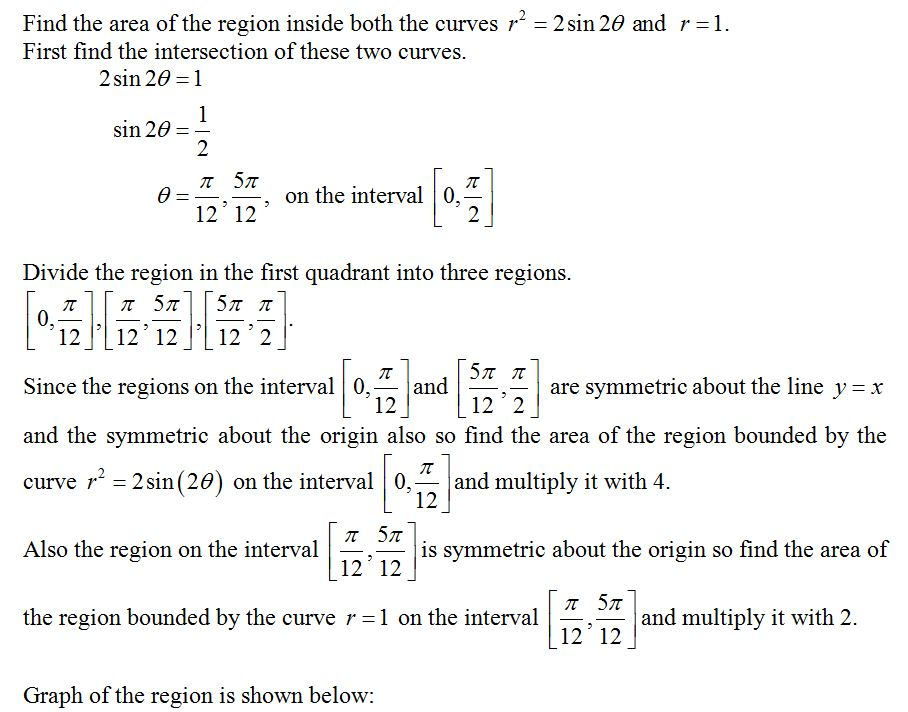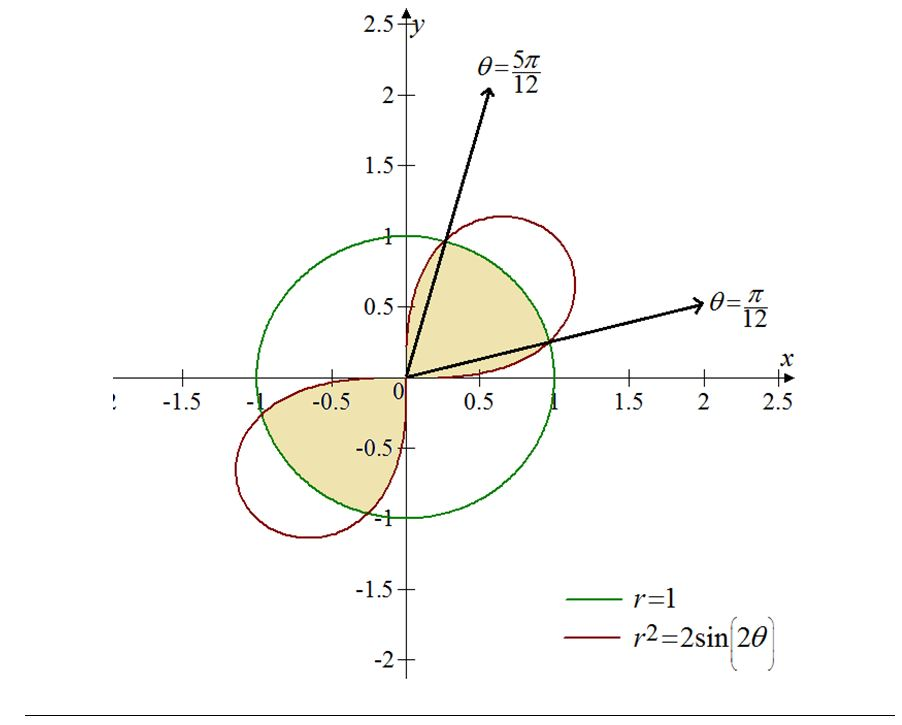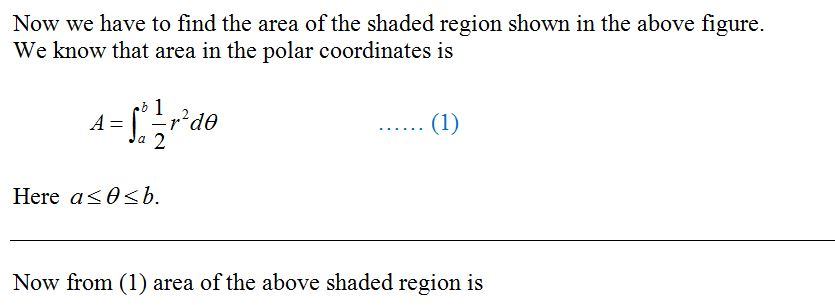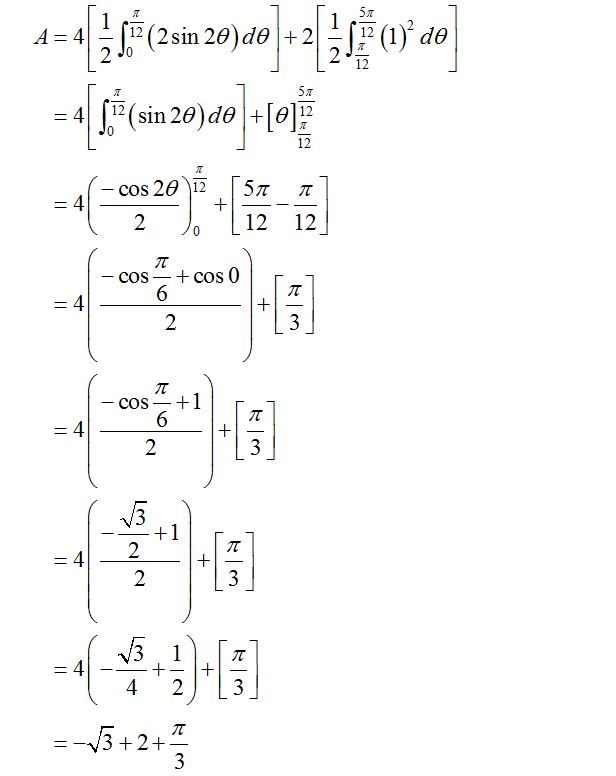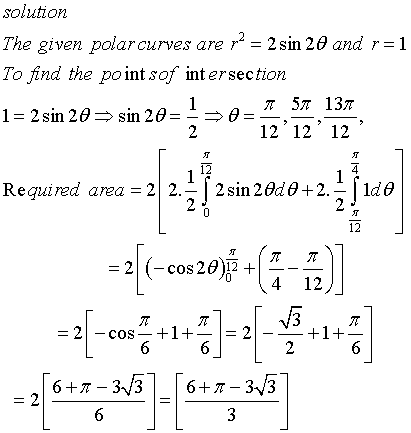# Find the area of the region that lies inside both curves. r2 = 2 sin(2θ). r = 1

Find the area of the region that lies inside both curves. r2= 2 sin(2θ), r =
1
r 2 = 2 sin(2 θ ), r =
1

Find the area of the region inside both the curves r2-2 sin 2θ and r = 1. First find the intersection of these two curves. 2 sin 20 -1 sin 2θ = 2 on the interval 0, 2 12 12 Divide the region in the first quadrant into three regions 1212 12 12 2 Since the regions on the interval 0,and and the symmetric about the origin also so find the area of the region bounded by the curver2 2sin (20) on the interval 0, and multiply it with 4. are symmetric about the line y-x 12 12’2 12 Also the region on the interval is symmetric about the origin so find the area of 12 12 the region bounded by the curve r1 on the interval and multiply it with 2. 12 12 Graph of the region is shown below:
2.5 12 1.5 0.5 12 0.5 0 0.5 1.5 2 2.5 0.5 1.5 1 2
Now we have to find the area of the shaded region shown in the above figure. We know that area in the polar coordinates is A- Ja Here a θ b. Now from (1) area of the above shaded region is
12 12 cos 28)12 し12 12 COS+ cos 0 2 COS1 2 2 2 4 23 –J3 + 2 +

solution The given polar curves are r-2sn2θ and r To find the point sof int ersection =1 12 12 12 12 – COS 0 412 647-3J3